Next: Electrostatic Fields Up: Maxwell's Equations Previous: Electromagnetic Momentum Conservation

# Exercises

1. Demonstrate that the energy contained in the magnetic field generated by a stationary current distribution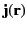in vacuum is given by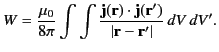2. A transverse plane wave is incident normally in vacuum on a perfectly absorbing flat screen. Show that the pressure exerted on the screen is equal to the electromagnetic energy density of the wave.

3. Consider an infinite parallel-plate capacitor. Let the lower plate lie at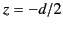, and carry the charge density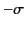. Likewise, let the upper plate lie at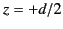, and carry the charge density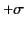. Calculate the electromagnetic momentum flux across the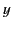-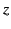plane. Hence, determine the direction and magnitude of the force per unit area that the plates exert on one another.

4. The equation of electromagnetic angular momentum conservation takes the general form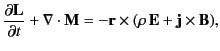where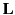is the electromagnetic angular momentum density, and the tensor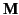is the electromagnetic angular momentum flux. Demonstrate that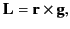and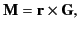where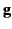is the electromagnetic momentum density, and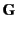the electromagnetic momentum flux tensor.

5. A long solenoid of radius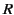, with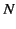turn per unit length, carries a steady current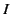. Two hollow cylinders of length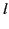are fixed coaxially such that they are free to rotate. The first cylinder, whose radius is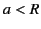, carries the uniformly distributed electric charge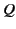. The second cylinder, whose radius is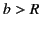, carries the uniformly distributed electric charge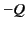. Both cylinders are initially stationary. When the current is switched off the cylinders start to rotate. Find the final angular momenta of the two cylinders, and demonstrate that the total angular momentum of the system is the same before and after the current is switched off.

6. Consider a system consisting of an electric chargeand a magnetic monopole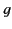separated by a distance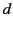. Demonstrate that the total angular momentum stored in the resulting electromagnetic fields is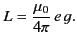[Hint: The radial magnetic field generated a distance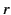from a magnetic monopole of strengthis of magnitude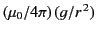.]Next: Electrostatic Fields Up: Maxwell's Equations Previous: Electromagnetic Momentum Conservation
Richard Fitzpatrick 2014-06-27